# Quantity based Quiz for IBPS , SBI , RBI: Quiz – 4

## Quantity based Quiz for IBPS , SBI , RBI: Quiz – 4

Quantity Based Questions are one of the topics of Quantitative Aptitude Section that is commonly asked in competitive exams. Candidates must have a clear understanding of Quantity Based Questions. In this article, we have come up with the Quantity Based Quiz to enhance your preparation. Each question in this Quantity Based Quiz has a full explanation. This Quantity Based Quiz is based on the current or updated exam pattern. This Quantity Based Quiz is free of cost. This Quantity Based Quiz contains questions that range in difficulty from easy to tough. Candidates must solve this Quantity Based Quiz to boost their preparation for upcoming exams.

Directions (1-10): In the given questions, two quantities are given, one as Quantity I and another as Quantity II. You have to determine relationship between two quantities and choose the appropriate option.

1. Number of ways in which word ‘SOMETHING’ can be arranged.
Quantity I : When all vowels comes together.
Quantity II: When all consonants come together.
(a) Quantity I > Quantity II
(b) Quantity I < Quantity II
(c) Quantity I ≥ Quantity II
(d) Quantity I ≤ Quantity II
(e) Quantity I = Quantity II or No relation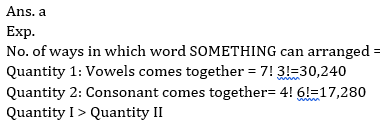2. A certain sum of money amounts to Rs. 2613 in 6 years at 5% per annum.
Quantity I: No. of years in which the same amount becomes Rs. 3015 at same rate.
Quantity II: 11 years.
(a) Quantity I > Quantity II
(b) Quantity I < Quantity II
(c) Quantity I ≥ Quantity II
(d) Quantity I ≤ Quantity II
(e) Quantity I = Quantity II or No relation3. Quantity I : A sphere of diameter 13.4 cm is melted and cast into a right circular cone of height 26.8 cm. The radius of the base of the cone is ?
Quantity II: 5.95 cm.
(a) Quantity I > Quantity II
(b) Quantity II > Quantity I
(c) Quantity I ≥ Quantity II
(d) Quantity II ≥ Quantity I
(e) Quantity I = Quantity II or relation can’t be established.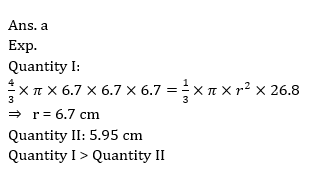4. A, B and C complete a task and receive Rs. 8880. They work for equal number of days. Five times of A’s efficiency is equal to four times of B’s efficiency which is equal to six times of C’s efficiency.
Quantity I: 2400
Quantity II: Amount received by C.
(a) Quantity I > Quantity II
(b) Quantity I < Quantity II
(c) Quantity I ≥ Quantity II
(d) Quantity I ≤ Quantity II
(e) Quantity I = Quantity II or No relation5. A solution of acid and water contains 20% acid in it.
Quantity I: Percentage of water that must be evaporated from solution to get a 50% acid solution.
Quantity II: 80%
(a) Quantity I > Quantity II
(b) Quantity I < Quantity II
(c) Quantity I ≥ Quantity II
(d) Quantity I ≤ Quantity II
(e) Quantity I = Quantity II or No relation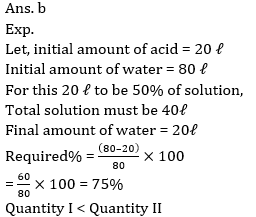6. A person lends ⅓rd of his money at 15% while the rest at 18% per annum rate at simple interest.
Quantity I: His annual rate of interest on the whole sum.
Quantity II: 16%.
(a) Quantity I > Quantity II
(b) Quantity I < Quantity II
(c) Quantity I ≥ Quantity II
(d) Quantity I ≤ Quantity II
(e) Quantity I = Quantity II or No relation7. Quantity I : curved surface area of a cone.
If the base radius of cone is 8 cm & height is 25% less than its radius.
Quantity II: Curved surface area of a cylinder.
If maximum volume of cylinder is 200π and its height is 60% more than its radius.
(a) Quantity I > Quantity II
(b) Quantity II > Quantity I
(c) Quantity I ≥ Quantity II
(d) Quantity II ≥ Quantity I
(e) Quantity I = Quantity II or relation can’t be established.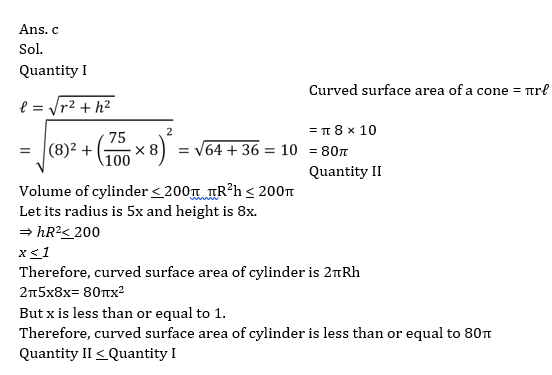8. Vinit, Suraj and Dinesh can do a work in 48 days, 36 days and 32 days respectively.
Quantity I – If Aarav is 100/3% more efficient than Dinesh, then find in how many days Aarav and Suraj will complete the same work together.
Quantity II – 77/5days
(a) Quantity I = Quantity II
(b) Quantity I > Quantity II
(c) Quantity I ≥ Quantity II
(d) Quantity I < Quantity II
(e) Quantity I ≤ Quantity II9. Quantity I – 5x² + 23x + 18 = 0
Quantity II – 3y² + 4y + 1 = 0
(a) Quantity I ≥ Quantity II
(b) Quantity I ≤ Quantity II
(c) Quantity I = Quantity II
(d) Quantity I < Quantity II
(e) Quantity I > Quantity II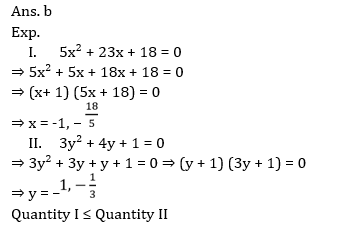10. A, B & C started a business by making investment in the ratio of 5 : 4 : 6 for 8 months, 6 months and 4 months respectively.
Quantity I – If difference between profit share of A and C is Rs. 450, then 60% of profit share of B.
Quantity II – Rajeev has Rs. 675. He gives 25% of that, to his child and 20% of remaining to his wife, what amount Rajeev kept with himself.
(a) Quantity I ≤ Quantity II
(b) Quantity I > Quantity II
(c) Quantity I = Quantity II
(d) Quantity I < Quantity II
(e) Quantity I ≥ Quantity II#### Attempt Quantitative Aptitude Topic Wise Online Test Series

Recommended PDF’s for:

#### Most important PDF’s for Bank, SSC, Railway and Other Government Exam : Download PDF Now

AATMA-NIRBHAR Series- Static GK/Awareness Practice Ebook PDF Get PDF here
The Banking Awareness 500 MCQs E-book| Bilingual (Hindi + English) Get PDF here
AATMA-NIRBHAR Series- Banking Awareness Practice Ebook PDF Get PDF here
Computer Awareness Capsule 2.O Get PDF here
AATMA-NIRBHAR Series Quantitative Aptitude Topic-Wise PDF Get PDF here
AATMA-NIRBHAR Series Reasoning Topic-Wise PDF Get PDF Here
Memory Based Puzzle E-book | 2016-19 Exams Covered Get PDF here
Caselet Data Interpretation 200 Questions Get PDF here
Puzzle & Seating Arrangement E-Book for BANK PO MAINS (Vol-1) Get PDF here
ARITHMETIC DATA INTERPRETATION 2.O E-book Get PDF here

3